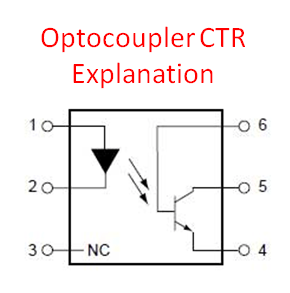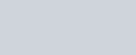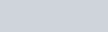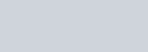# Opto Coupler CTR Explanation

Opto coupler CTR explanation is not well elaborated by other authors and before I was also having hard time of understanding what is it. Current transfer ratio or commonly known as CTR is the ratio of the collector current to the forward current of an optocoupler. It is synonymous to a current gain of a bipolar junction transistor. If this is the first time you heard about optocoupler, it is recommended that you will read How Optocoupler Works.

CTR is expressed in terms of percentage as below:Wherein Ic is the collector current while If is the forward current.

The device CTR is specified in the datasheet. As long as the operation of the optocoupler circuit is in the linear region, the forward and the collector current are always linked by its other through the component CTR. However, once the optocoupler saturates, the circuit CTR is no longer the same with the device CTR and the collector current cannot be computed anymore using the equation above.

Supposing at linear operation the forward current is 5mA and the CTR of the device specified in the datasheet is 100% (typical), therefore the collector current will be 5mA too. When the operation is saturation, the collector current is cannot be computed using the same approach. At saturation, the circuit is no longer gaining and the collector current is only dependent to the collector limiting resistor.

To explain further, consider the circuit in Figure 1. Once the circuit operation is linear, the collector current Ic is just the product of the forward current If and the device CTR.This means that the value of R2 is nothing to do with the collector current at linear operation. However, the value of R2 will affect the collector-emitter voltage.

At saturation, you cannot use anymore the CTR equation in computing the collector current Ic. At this condition, Ic is only dependent to the value of the resistor R2.## Factors Affecting Device CTR

Device current transfer ratio is dependent to the luminous intensity of the light source, operating temperature and continuous operation or aging.

### Opto Coupler CTR Explanation : Luminous Intensity of the Light Source

This is dependent to the forward current applied to the light source which is commonly a light emitting diode. The higher the forward current, the brighter is the light and the higher the CTR will be. However, operating at higher forward current will degrade the life of the optocoupler faster, so better consider it as well. Figure 2 shows an example graph of forward current versus the current transfer ratio of a particular optocoupler device. As you can see, at 1mA the CTR is around 100% while it increases to more than 200% when the forward current is reaching 10mA for a VCE of 5V. You have to take note also not to operate in much higher current because it is not anymore helpful in you design since the CTR will start to decrease at some point based from the graph.

### Opto Coupler CTR Explanation : Ambient Temperature Dependency

The luminous efficiency of the LED has a negative temperature coefficient while a positive coefficient for the photo transistor HFE (Figure 3). This will result to a curve shown in Figure 4.

If your design is exposed in a higher temperature, say 100’C, the corresponding CTR will de-rate to 60% of the initial value.

### Aging

Continuous operation of the opto-coupler will decrease its life expectancy because the luminous efficiency of the light source will degrade over time. Another factor is that the optical coupling efficiency between the LED and the photo-transistor will degrade over time. For the photo transistor, the photo-electrical conversion efficiency and the DC amplification factor will be affected by continuous operation.

Figure 5 shows a graph between operating time and relative CTR. As you can see, the CTR starts to de-rate after 20,000 hours.

### Example Device CTR Calculation

To complete the discussion, I will demonstrate how to get the device CTR with forward current, operating temperature and aging consideration.

Considering a 5mA forward current, 50’C operating temperature and 100,000 hours life.

From Figure 2 the corresponding CTR is 200% considering 5V VCE. From Figure 4 the de-rating due to temperature is around 90%. The CTR de-rating after 100,000 hours of operation is around 85% based from Figure 5. The resulting device CTR is